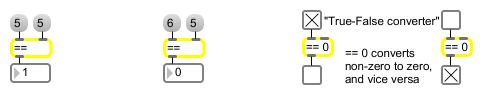# ==

Compare two numbers, output 1 if they are equal

## Description

== compares two given numbers, if they are equal, == outputs a 1, if they are not equal, == outputs a 0.

## Arguments

Name Type Opt Description
initial-comparison-value int or float opt Sets the initial value, to be compared with a number received in the left inlet. A float argument forces a float comparison.

## Messages

 bang In left inlet: Performs the comparison with the numbers currently stored. If there is no argument, == initially holds 0 for comparison. int input [int] In left inlet: The number is compared with the number in the right inlet. If the two numbers are equal, == outputs 1. If they are not equal == outputs 0. (inlet1) comparison-value [int] In right inlet: The number is stored to be compared with a number received in the left inlet. float input [float] Converted to int before comparison, unless == has a float argument. set input [int] Sets the number to be compared without causing output (bang will output it). list input and comparison-value [list] In left inlet: Compares first and second number, outputs 1 if they are equal, 0 if they are not equal.

## Output

int: 1 if the numbers in the inlets are equal, 0 if they are not equal.

## ExamplesThe numbers are equal...... The numbers are not equal..... Using == 0 as a logical 'not'